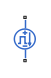# Pulse Current Source

Periodic square wave current source

• Library:
• Simscape / Electrical / Sources

Simscape / Electrical / Additional Components / SPICE Sources

•## Description

The Pulse Current Source block represents a current source whose output current value is a periodic square pulse as a function of time and is independent of the voltage across the terminals of the source. The following equations describe the current through the source as a function of time:

`${I}_{out}\left(0\right)={I}_{1}$`

`${I}_{out}\left(TD\right)={I}_{1}$`

`${I}_{out}\left(TD+TR\right)={I}_{2}$`

`${I}_{out}\left(TD+TR+PW\right)={I}_{2}$`

`${I}_{out}\left(TD+TR+PW+TF\right)={I}_{1}$`

`${I}_{out}\left(TD+PER\right)={I}_{1}$`

Where:

• I1 is the output current at time zero.

• I2 is the output current when the output is high.

• TD is the time at which the pulse first starts.

• TR is the time that it takes the output current to rise from I1 to I2.

• TF is the time it takes the output current to fall from I2 to I1.

• PW is the time width of the output pulse.

• PER is the period of the output pulse.

The block determines the values at intermediate time points by linear interpolation.

The specified values for PW and PER have the following effect on the block output:

• If both PW and PER are infinite, the block produces a step response at time TD.

• If PER is infinite and PW is finite, the block produces a single pulse of width PW and infinite period.

• If PW is infinite and PER is finite, the block produces a step response with pulses of width TR to a value I1 every PER seconds.

• If PW > PER, the block produces a step response with pulses of width TR to a value I1 every PER seconds.

The block uses a small conductance internally to prevent numerical simulation issues. The conductance connects the + and - ports of the device and has a conductance GMIN:

• By default, GMIN matches the GMIN parameter of the Environment Parameters block, whose default value is `1e–12``1/Ohm`.

• To change GMIN, add an Environment Parameters block to your model and set the GMIN parameter to the desired value.

## Ports

### Conserving

expand all

Electrical conserving port associated with the pulse current source positive terminal.

Electrical conserving port associated with the pulse current source negative terminal.

## Parameters

expand all

Value of the output current at time zero, in A.

Value of the output current when the output is high, in A.

Time at which the pulse first starts, in s.

Time it takes the output current to rise from the Initial value, I1 value to the Pulse value, I2 value. The value must be greater than or equal to `0`.

Time it takes the output current to fall from the Pulse value, I2 value to the Initial value, I1 value. The value must be greater than or equal to `0`.

Time width of the output pulse. The value must be greater than `0`.

Period of the output pulse. For the default value, `Inf``s`, the block produces a single pulse with an infinite period.

Specify if the current source includes a parallel conductance. The value of the parallel conductance, GMIN, depends on whether your model contains an Environment Parameters block.

This table shows the possible values of the parallel conductance, GMIN:

Values of parallel conductance, GMIN

Include parallel conductance, GMIN
`Yes``No`
Model contains Environment Parameters blockModel does not contain Environment Parameters blockParallel conductance, GMIN, is not included and it does not affect the current source.
GMIN is equal to the value of the GMIN parameter in the Environment Parameters block.GMIN = `1e-12` `Ohm`

## Version History

Introduced in R2008a